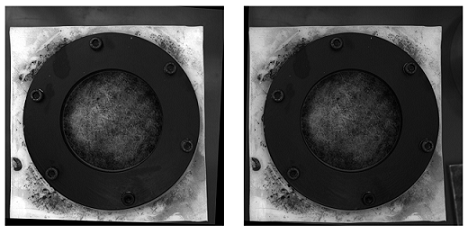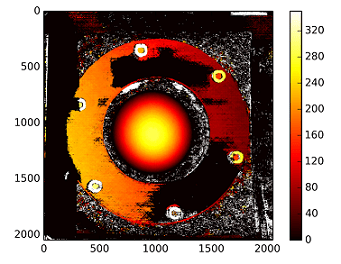# #StackBounty: #python #opencv #stereo-3d #disparity-mapping OpenCV – Depth map from Uncalibrated Stereo System

### Bounty: 500

I’m trying to get a depth map from an uncalibrated method.
I can obtain the fundamental matrix via different correspondent points from SIFT method and `cv2.findFundamentalMat`. Then with `cv2.stereoRectifyUncalibrated` I can get the rectification matrix. Finally I can use `cv2.warpPerspective` to rectify and compute the disparity but this latter doesn’t conduct to a good depth map. The values are very high so I’m wondering if I have to use `warpPerspective` or I have to calculate rotation matrix from homography matrix got with `stereoRectifyUncalibrated`.

I’m not sure of the projective matrix with the case of homography matrix obtained with the `stereoRectifyUncalibrated` to rectify.

A part of the code :

``````#Obtainment of the correspondent point with SIFT
sift = cv2.SIFT()

###find the keypoints and descriptors with SIFT
kp1, des1 = sift.detectAndCompute(dst1,None)
kp2, des2 = sift.detectAndCompute(dst2,None)

###FLANN parameters
FLANN_INDEX_KDTREE = 0
index_params = dict(algorithm = FLANN_INDEX_KDTREE, trees = 5)
search_params = dict(checks=50)

flann = cv2.FlannBasedMatcher(index_params,search_params)
matches = flann.knnMatch(des1,des2,k=2)

good = []
pts1 = []
pts2 = []

###ratio test as per Lowe's paper
for i,(m,n) in enumerate(matches):
if m.distance < 0.8*n.distance:
good.append(m)
pts2.append(kp2[m.trainIdx].pt)
pts1.append(kp1[m.queryIdx].pt)

pts1 = np.array(pts1)
pts2 = np.array(pts2)

#Computation of the fundamental matrix

# Obtainment of the rectification matrix and use of the warpPerspective to transform them...

pts1 = np.int32(pts1)
pts2 = np.int32(pts2)

p1fNew = pts1.reshape((pts1.shape * 2, 1))
p2fNew = pts2.reshape((pts2.shape * 2, 1))

retBool ,rectmat1, rectmat2 = cv2.stereoRectifyUncalibrated(p1fNew,p2fNew,F,(2048,2048))

dst11 = cv2.warpPerspective(dst1,rectmat1,(2048,2048))
dst22 = cv2.warpPerspective(dst2,rectmat2,(2048,2048))

#calculation of the disparity
disp = stereo.compute(dst22.astype(uint8), dst11.astype(uint8)).astype(np.float32)
plt.imshow(disp);plt.colorbar();plt.clim(0,400)#;plt.show()
plt.savefig("0gauche.png")

#plot depth by using disparity focal length `C1[0,0]` from stereo calibration and `T` the distance between cameras

plt.imshow(C1[0,0]*T/(disp),cmap='hot');plt.clim(-0,500);plt.colorbar();plt.show()
``````

Here the rectified pictures with uncalibrated method (and `warpPerspective`) :Here the rectified pictures with calibrated method :I don’t know how the difference is so important between the two kind of pictures…and for the calibrated method, it doesn’t seem aligned…strange
The disparity map of the uncalibrated method :And the depth map are calculated with : `C1[0,0]*T/(disp)`
with T from the `stereoCalibrate` but the values are very high…

——– EDIT LATER ————

I tried to "mount" the reconstruction matrix ([Devernay97], [Garcia01]) with the homography matrix obtained with the "stereoRectifyUncalibrated" but the result are not good… Is my use correct?

``````Y=np.arange(0,2048)
X=np.arange(0,2048)
(XX_field,YY_field)=np.meshgrid(X,Y)

#I mount the X, Y and disparity in a same 3D array
stock = np.concatenate((np.expand_dims(XX_field,2),np.expand_dims(YY_field,2)),axis=2)
XY_disp = np.concatenate((stock,np.expand_dims(disp,2)),axis=2)

XY_disp_reshape = XY_disp.reshape(XY_disp.shape*XY_disp.shape,3)

Ts = np.hstack((np.zeros((3,3)),T_0)) #i use only the translations obtained with the rectified calibration...Is it correct?

# I establish the projective matrix with the homography matrix
P11 = np.dot(rectmat1,C1)
P1 = np.vstack((np.hstack((P11,np.zeros((3,1)))),np.zeros((1,4))))
P1[3,3] = 1

# P1 = np.dot(C1,np.hstack((np.identity(3),np.zeros((3,1)))))

P22 = np.dot(np.dot(rectmat2,C2),Ts)
P2 = np.vstack((P22,np.zeros((1,4))))
P2[3,3] = 1

lambda_t = cv2.norm(P1[0,:].T)/cv2.norm(P2[0,:].T)

#I define the reconstruction matrix
Q = np.zeros((4,4))

Q[0,:] = P1[0,:].T
Q[1,:] = P1[1,:].T
Q[2,:] = lambda_t*P2[1,:].T - P1[1,:].T
Q[3,:] = P1[2,:].T

#I do the calculation to get my 3D coordinates
test = []
for i in range(0,XY_disp_reshape.shape):
a = np.dot(inv(Q),np.expand_dims(np.concatenate((XY_disp_reshape[i,:],np.ones((1))),axis=0),axis=1))
test.append(a)

test = np.asarray(test)

XYZ = test[:,:,0].reshape(XY_disp.shape,XY_disp.shape,4)
``````

Get this bounty!!!

This site uses Akismet to reduce spam. Learn how your comment data is processed.